×#### Thank you for registering.

One of our academic counsellors will contact you within 1 working day.

Click to Chat

1800-1023-196

+91-120-4616500

CART 0

• 0

MY CART (5)

Use Coupon: CART20 and get 20% off on all online Study Material

ITEM
DETAILS
MRP
DISCOUNT
FINAL PRICE
Total Price: Rs.

There are no items in this cart.
Continue Shopping```Limits, Continuity and Differentiability

Table of Content

What is the concept of limit?

What do we mean by the continuity of a function?

Discontinuity

What do we mean by the differentiability of a function?

Related ResourcesLimits, Continuity and Differentiability can in fact be termed as the building blocks of Calculus as they form the basis of entire Calculus.

The basic concept of limit of a function lays the groundwork for the concepts of continuity and differentiability. These concepts can in fact be called the natural extensions of the concept of limit. In this section we shall just give a brief outline of all these three concepts as they have been discussed in detail in the coming sections. This is well illustrated through carefully selected solved problems of different levels.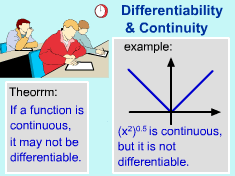"Limits, Continuity and Differentiability" is one of the easiest and important chapters of Calculus in the Mathematics syllabus of IIT JEE, AIEEE and other  engineering examinations. These laws are not only important from the point of view of Mathematics but they are also very useful to a large extent in Physics and  Physical Chemistry. The examples based on this are very easy and can be helpful in mastering the topic.

The chapter is important not only because it fetches 3-4 questions in most of the engineering examination but also because it is prerequisite to the other chapters of Calculus. So, said that, let’s begin our journey of limits followed by continuity and differentiability:

What is the concept of limit?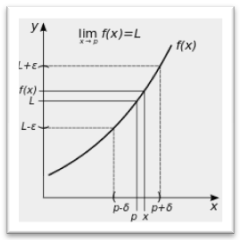Let f be a function defined on an open interval containing a point ‘p’ (except possibly at p) and let us assume ‘L’ to be a real number.

Then, the function f is said to tend to a limit ‘L’ written as lim x→p f(x) = L if for each ∈ > 0, there exists a δ > 0 such that for all x satisfying 0 < |x – p| < δ, we have |f(x) – L| < ∈ or symbolically, we can define it as

∀ ∈ > 0, ∃ a δ > 0 such that 0 < |x – p| < δ ⇒ |f(x) – L| < ∈.

The concept of limits can further be divided into two heads which are illustrated by the below diagram:Let y = f(x) be a given function and x = a is the point under consideration. Left tendency of f(x) at x = a is called its left limit and right tendency is called its right limit.

Left tendency (left limit) is denoted by f(a - 0) or f(a-) and right tendency (right limit) is denoted by f(a + 0) or f(a+) and are written as

f(a – 0) = lim h→0 f(a – h)

f(a + 0) = lim h→0 f(a + h), where 'h' is a small positive number.

Thus for the existence of the limit of f(x) at x = a, it is necessary and sufficient that f(a – 0)  = f(a + 0), if these are finite or f(a – 0) and f(a + 0) both should be either +∞ or -∞.

These limits have been discussed in detail in the coming pages but the key point to note here is thatLimit of a function exists at a point ‘a’ iff both the left and right hand limits exist and are equalSeveral methods can be used for evaluating the limit of a function. We have just listed the various methods here. For more information on the methods, students are advised to refer the later sections.Direct Substitution Method

Factorization Method

Rationalization

Evaluation using Standard Limits

Limits at infinity

Using Sandwich theorem

What do we mean by the continuity of a function?A function f(x) is said to be continuous at x = a if

lim x→a- f(x) = lim x→a+ f(x) = f(a).

Thus, unlike limits, for continuity it is essential for the function to be defined at that particular point and the limiting value of the function should be equal to f(a).

The sum, difference, product and quotient of two continuous functions is always continuous. But, in case of quotient of two functions, the denominator must be zero.

A function is said to be discontinuous in any of the following cases:

1. lim x→a- f(x) and lim x→a+ f(x) exist but are not equal.

2. lim x→a- f(x) and lim x→a+ f(x) exist and are equal but not equal to f(a).

3. f(a) is not defined.

4. At least one of the limits does not exist.A function is continuous when its graph is a single unbroken curve …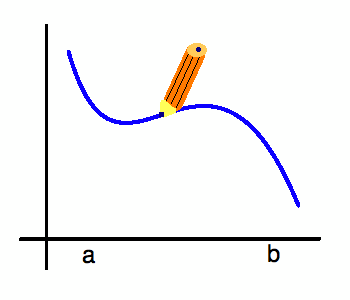….. if you can draw the curve without lifting your hand from the paper, the curve is continuous.

Continuity of a function in an interval:

If f is continuous in [a, b] then in addition to being continuous at every point of domain, f should also be continuous at the end points i.e. f(x) is said to be continuous in the closed interval [a, b] if

f(x) is continuous in (a, b)

lim x→a+ f(x) = f(a)

lim x→a- f(x) = f(a)

Discontinuity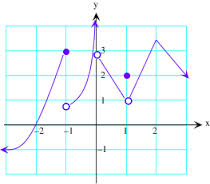We have already discussed the case when a function is termed to be discontinuous. A function may be discontinuous if either the left hand limit or the right hand limit does not exist or if they exist then the value is not equal to the value of the function. The possible types of discontinuities are listed below:

We can classify the types of discontinuities into two broad categories:

Removable discontinuity and Non-Removable discontinuity

These can further be classified as under: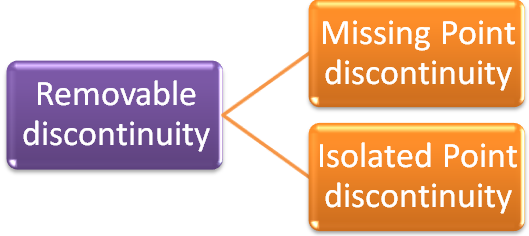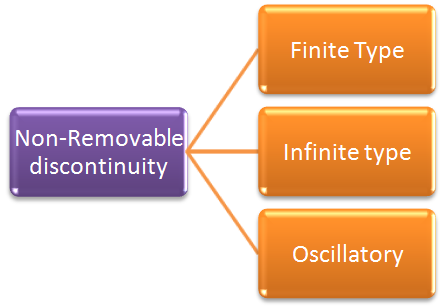What do we mean by the differentiability of a function?Derivability or differentiability of a function has a twofold meaning:

Geometrical Meaning of derivative

Physical Meaning of derivative

The derivative of f, denoted by f'(x) is given by

f'(x) = lim ?x→0 (? y)/(? x) = dy/dx

A function f is said to be derivable over a closed interval [a, b] if :

For the points a and b, f'(a+) and f'(b-) exist and

For ant point c such that a < c < b, f'(c+) and f'(c-) exist and are equal.Differentiability always implies continuity but the converse is not true. This means that a differentiable function is always continuous but if a function is continuous it may or may not be differentiable.

Let us now have a look at a question on differentiability once asked in IIT JEE. For in-depth study of the topic, students may refer the later sections on the topic.

Illustration 1:

Let [.] denotes the greatest integer function and f(x) = [tan2x], then does the limit exist or is the function differentiable or continuous at 0? (IIT JEE 1999)

Solution:

Given f(x) = [tan2x]

Now, -45°< x < 45°

tan (-45°) < tan x < tan 45°

-tan 45°< tan x < tan 45°

-1 < tan x < 1

So, 0 < tan2x < 1

[tan2x] = 0

So, f(x) is zero for all values of x form x = -45° to 45°.

Hence, f is continuous at x = 0 and f is also differentiable at 0 and has a value zero.

Limits, Continuity and Differentiability is important from the perspective of scoring high in IIT JEE as there are few fixed patterns on which a number of Multiple Choice Questions are framed on this topic. You are expected to do all the questions based on this to remain competitive in IIT JEE examination. It is very important to master these concepts at early stage as this forms the basis of your preparation for IIT JEE and other engineering entrance examinations.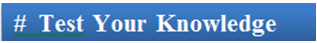Q1. The relationship between limit and continuity of a function is

(a) existence of limit guarantees the continuity of the function

(b) left limit will imply that the function is continuous

(c) if both the left and right hand limits exist and are equal to the value of the function at the particular point then the function is said to be continuous at that point.

(d) none of these

Q2. Suppose the left and right derivatives of a function exist but are not equal. Then

(a) the function is not differentiable

(b) the function is differentiable because the derivatives exist

(c) the function may or may not be differentiable

(d) none of these

Q3. If the limit of a function at a point a exists but is not equal to the value of the function at that point, then

(a) existence of limit guarantees the continuity of the function

(b) the function is said to have removable discontinuity

(c) if both the left and right hand limits exist and are equal to the value of the function at the particular point then the function is said to be continuous at that point.

(d) none of these

Q4. The types of discontinuities can be basically classified into two broad categories namely:

(a) left and right discontinuity.

(b) upper and lower discontinuity

(c) first order and second order discontinuity

(d) removable and non-removable discontinuities.

Q5. Continuity and differentiability are inter-related as

(a) a differentiable function is obviously continuous.

(b) continuity implies differentiability.

(c) a function is continuous iff it is differentiable.

(d) none of theseQ1.

Q2.

Q3.

Q4.

Q5.

(c)

(a)

(b)

(d)

(a)

Related Resources

You may wish to refer Limits using Sandwich Theorem.

For getting an idea of the type of questions asked, refer the previous year papers.

To read more, Buy study materials of Limtis and Continuity comprising study notes, revision notes, video lectures, previous year solved questions etc. Also browse for more study materials on Mathematics here.

```### Course Features

• 731 Video Lectures
• Revision Notes
• Previous Year Papers
• Mind Map
• Study Planner
• NCERT Solutions
• Discussion Forum
• Test paper with Video Solution